# How to remove a specific element from queue

## Introduction

A queue is an abstract data type generally used in the same way as the name suggests.

In Queue, the insertion of elements occurs at the rear end, and deletion occurs at the front end, works in (FIFO) manner.

Inserting in the Queue in the rear end is known as Enqueue, and Deleting from the Queue from the front end is called Deque. Queue supports access to the elements in the Queue from both ends of the structure.

In this article, we’ll learn how to delete a particular element from a queue.

## Problem Statement

Given a queue and an integer k, find a method to delete the element k from the Queue and print the Queue after deleting.

Note: If there is more than one occurrence of k in the Queue, delete the first occurrence only. If k is not present in the Queue, print that k doesn’t exist in the Queue.

For example:

INPUT : q = [2,1,3,4,5,], k = 4

OUTPUT:  [2,1,3,5]

4 occurs only once in the queue so it’s deleted and then the queue is [2,1,3,5].

INPUT : q = [2,1,4,3,4,5], k=4

OUTPUT:  [2,1,3,4,5]

4 occurs twice in this queue. So, we delete the first occurrence at index 2, and thus the queue is [2,1,3,4,5].

## Solution Approach

The solution to this problem is quite simple. It’s just the implementation.

What we will do is, we will keep popping out the elements from the Queue until we find the first occurrence of k. We don’t want to lose other elements. Therefore, we will keep storing the popped elements in a vector. Once k is found, we will pop it out from the Queue, and then we will pop the rest of the elements and keep storing them in the vector.

Note that the first occurrence of k is not there in the vector. So basically, the vector contains all the elements other than the first occurrence of k. We just need to push the elements of the vector into the Queue. Then the Queue will contain all the elements other than the first occurrence of k.

Steps are:

• Input the number of elements in the Queue
• input the numbers to be added to the Queue
• Add those numbers to the Queue
• Input the value of k
• Declare and initialize a found variable which will be 0 if the first occurrence of k is not found; otherwise, 1
• Declare the vector v for storing the numbers after popping out
• If the current front of the Queue is k and we have not found it earlier, i.e., found==0 then, this is the first occurrence. So we pop it out and don't insert it into the vector
• Update the value of found to 1 as we have found the first occurrence of k in this case
• Else, push the current front into the vector to store it and pop it from the Queue
• Check if k is found yet. If not, print that k is not present in the Queue.
• Else, now, the vector contains all the elements other than the first occurrence of k, and the Queue is empty. So, we push all the elements of the vector into the Queue one by one
• Now, the Queue contains all the elements other than the first occurrence of k in the correct order. We print these elements one by one from the Queue.

## Implementation

The implementation of the above approach is given in the following three languages.

### C++ Implementation

Below is the C++ implementation of the above-discussed approach.

``````#include <bits/stdc++.h>
using namespace std;

int main()
{
int n;
cout<<"Enter the number of elements in the queue: ";
cin >> n; // Input the number of elements in the queue
queue<int> q;
cout<<"Enter elements one by one: ";
for (int i = 0; i < n; i++)
{
int x;
cin >> x;  // input the numbers to be added in the queue
q.push(x); // Add those numbers in the queue
}
cout<<"Enter the element you want to remove: ";
int k;
cin >> k;      // Input the number which is to be removed
int found = 0; // Declare and initialise a found variable which will be 0 if the
vector<int> v; // Declare the vector v for storing the numbers after popping out
while (!q.empty())
{
if (q.front() == k && found == 0)
{ // If the current front of the queue is k and
// we have not found it earlier, i.e. found==0 then, this is the first
// occurrence. So we pop it out and don't insert into the vector
q.pop();
found = 1; // Update the value of found to 1 as we have found the first occurrence
// of k in this case
}
else
{ // Else, push the current front into the vector to store it and pop it from the queue
v.push_back(q.front());
q.pop();
}
}
if (found == 0)
{ // Check if k is found yet. If not, print that k is not present in the queue
cout << "K NOT PRESENT IN THE QUEUE" << endl;
}
// Else
else
{
// Now, the vector contains all the elements other than the first occurrence of k and
// queue is empty. So, we just push all the elements of the vector into the queue one by one
for (int i = 0; i < v.size(); i++)
{
q.push(v[i]);
}
// Now, the queue contains all the elements other than the first occurrence of k in the
// correct order.
// We just print these elements one by one from the queue.
while (!q.empty())
{
cout << q.front() << " ";
q.pop();
}
cout << endl;
}
}``````

#### Output: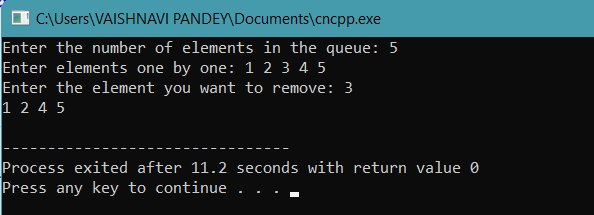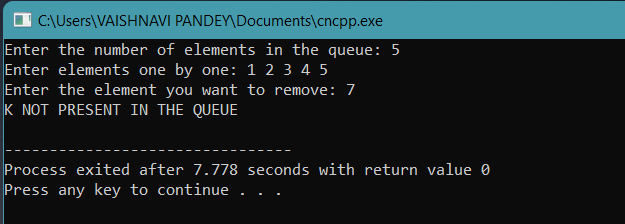### Java Implementation

Below is the Java implementation of the above-discussed approach.

``````import java.util.*;
public class RemoveElement{
public static void main(String[] args)
{
try (Scanner s = new Scanner(System.in))
{
System.out.println("Enter the number of elements:");
int n = s.nextInt(); // Input the number of elements in the queue
System.out.println("Enter the elements one by one:");
Queue < Integer > q = new LinkedList <> ();
for (int i = 0; i < n; i++)
{
int x = s.nextInt();
// input the numbers to be added in the queue
}
System.out.println("Enter the element to be removed");
int k = s.nextInt();
int found = 0;
ArrayList < Integer > v = new ArrayList <> ();
// Declare the array list v for storing the numbers after popping out
while (!q.isEmpty())
{
if (q.peek() == k && found == 0)
{ // If the current front of the queue is k and
// we have not found it earlier, i.e. found==0 then, this is the first
// occurrence. So we pop it out and don't insert into the vector
q.remove();
found = 1; // Update the value of found to 1 as we have found the first occurrence
// of k in this case
} else
{ // Else, push the current front into the vector to store it and pop it from the queue
q.remove();
}
}
if (found == 0) { // Check if k is found yet. If not, print that k is not present in the queue
System.out.println("K NOT PRESENT IN THE QUEUE");
}
// Else
else
{
// Now, the vector contains all the elements other than the first occurrence of k and
// queue is empty. So, we just push all the elements of the vector into the queue one by one
for (int i = 0; i < v.size(); i++)
{
}
// Now, the queue contains all the elements other than the first occurrence of k in the
// correct order.
// We just print these elements one by one from the queue.
while (!q.isEmpty()) {
System.out.print(q.peek() + " ");
q.remove();
}
System.out.println();
}
}
}
}``````

#### Output: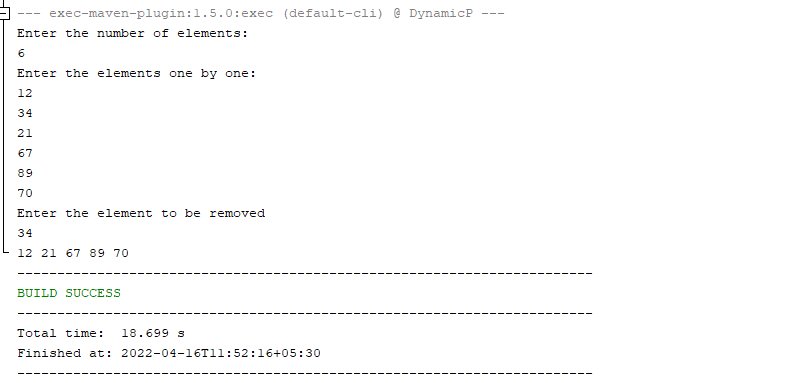### Python Implementation

Below is the Python implementation of the above-discussed approach.

``````class Node:
def __init__(self, data):
self.data = data
self.next = None

class Queue:
def __init__(self):
self.last = None
self.size = 0
# Create 'enqueue()' operation and pass 'self' and 'data' as parameters.

def enqueue(self, data):
new_node = Node(data)
if self.last is None:
else:
self.last.next = new_node
self.last = self.last.next
self.size += 1
# Create 'dequeue()' operation and pass 'self' as parameter.

def dequeue(self):
return None
else:
self.size -= 1
return temp

q = Queue()
n = int(input())

for i in range(n):
x = int(input())
q.enqueue(x)

temp = []
found = False

k = int(input())

while q.size != 0:
if q.head.data == k and found == False:
q.dequeue()
found = True
else:
q.dequeue()

if found == False:
else:
print(temp)``````

#### Output:

Entering the number of elements as 5 and elements as [ 6 2 4 3 5].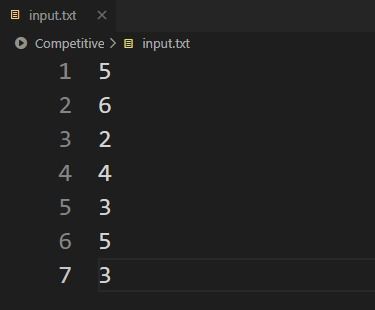And, the element to remove: 3

The final queue after removing 3 is: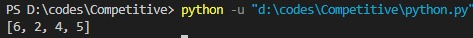## Time and Space Complexity

• Time complexity
O(n), where n is the number of elements in the Queue
Reason: We’ve only traversed the Queue and the vector once each. This only takes linear (O(n)) time.
• Space complexity
O(n), where n is the number of elements in the Queue
Reason: In the worst case, we might need to store all the elements in the vector v. That takes O(n) space.

1. What are Queue and their types in data structure?
A queue is a data structure that follows the property of first in, first out. There are four kinds of queues available – simple Queue, Circular Queue, priority queue, and double-ended Queue.

2. What are the types of queues?
We have two types of Queue: the circular Queue and the priority queue. The first item of the circular Queue is connected to the last to make a circle, and the elements of the priority queue are sorted based on priority.

3. What is the difference between stack and Queue?
Stack is only one end opened, so it follows the principle of Last in last out, and the Queue has both ends opened it follows First in first out principle.

4. How do I remove something from a queue?
To remove an element from a queue, bring that element to the front of the Queue and pop it out using the pop() function.

## Conclusion

In this article, we discussed how to delete a specific element from a queue. Problems involving the operations on queue data structure are quite popular. Some of these are: reversing a queuereverse the first k elements of a queue and implementation of Queue

You can try these out to gain more confidence on this topic. These questions are asked during various coding contests as well as placements tests.

To practice more such problems, Codestudio is a one-stop destination. This platform will help you acquire effective coding techniques and give you an overview of student interview experience in various product-based companies.

Happy Coding!